Lakhmir Singh & Manjit Kaur: Motion, Solutions- 2

# Lakhmir Singh & Manjit Kaur: Motion, Solutions- 2 - Science Class 9

Page No - 21

Question 28:
A tortoise moves a distance of 100 metres in 15 minutes. What is the average speed of tortoise in km/h ?
Solution :
Total distance=100m =0.1 km
Total time taken=15 minutes= 15/60=0.25 hour
Average speed = Total distance travelled/ Total time taken
=0.1/0.25= 0.4km/h

Question 29:
If a sprinter runs a distance of 100 metres in 9.83 seconds, calculate his average speed in km/h.
Solution :
Total distance travelled =100m
Total time taken = 9.83 sec
Average speed = Total distance travelled/ Total time taken
=100/9.83 =10.172m/s
Averge speed in km/h:
10.172 x(3600/1000)=36.62 km/h

Question 30:
A motorcyclist drives from place A to B with a uniform speed of 30 km h-1 and returns from place B to A with a uniform speed of 20 km h-1. Find his average speed.

Solution :

Speed from A to B = 30 km/h

Let the distance from A to B be D,

Time taken to travel from A to B,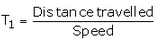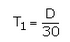Speed from B to A = 20 km/h

Time taken to travel from B to A, T2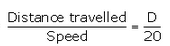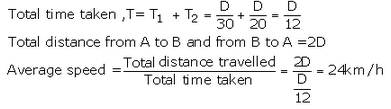Question 31:
A motorcyclist starts from rest and reaches a speed of 6 m/s after travelling with uniform acceleration for 3 s. What is his acceleration ?
Solution :
Initial velocity= 0m/s
Final velocity=6m/s
Time=3 sec
Initial Velocity = 0 m/s

Final Velocity = 6 m/s

Time = 3 Sec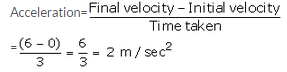Question 32:
An aircraft travelling at 600 km/h accelerates steadily at 10 km/h per second. Taking the speed of sound as 1100 km/h at the aircraft’s altitude, how long will it take to reach the ‘sound barrier’ ?
Solution :
Initial velocity, u = 600 km/h
Final velocity, v = 1100 km/h
Acceleration = 10 km/h/s = 600 km/h2
From relation, a = (v-u)/t
t = (v-u)/a
t = (1100-600)/600 = 500/600 = 5/6 hr = 50 sec

Question 33:
If a bus travelling at 20 m/s is subjected to a steady deceleration of 5 m/s2, how long will it take to come to rest ?
Solution :
Deceleration, a=-5m/s2
Initial velocity, u=20m/s
Final velocity, v=0m/s
t=?

Deceleration, a = -5m/s2

Initial Velocity, u = 20m/s

Final Velocity, v = 0 m/s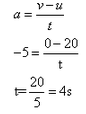Question 34:
(a) What is the difference between ‘distance travelled’ by a body and its ‘displacement’ ? Explain with the
help of a diagram.
(b) An ant travels a distance of 8 cm from P to Q and then moves a distance of 6 cm at right angles to PQ. Find its resultant displacement.
Solution :
(a) Distance travelled is the actual length of the indirect path covered by the body whereas displacement refers to the straight line path between the initial and final positions. For e.g. In the figure given below, a body moves from point A to point B and then from point B to point C. Here, the distance travelled by the body is AB + BC and displacement is AC.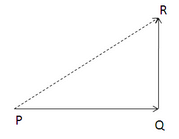PQ = 8cm

QR = 6 cm

Resultant Displacement PR =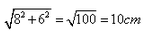Question 35:
Define motion. What do you understand by the terms ‘uniform motion’ and ‘non-uniform motion’ ? Explain with examples.
(a) Define speed. What is the SI unit of speed ?
(b) What is meant by (i) average speed, and (ii) uniform speed ?
Solution :
A body is said to be in motion when its position changes continuously with respect to a stationary object taken as reference point.
A body has uniform motion if it travels equal distances in equal intervals of time, no matter how small these time intervals may be. For example: a car running at a constant speed of 10m/s, will cover equal distance of 10m every second, so its motion will be uniform.
Non-uniform motion: A body has a non-uniform motion if it travels unequal distances in equal intervals of time. For example: dropping a ball from the roof of a tall building .

Question 36:
(a) Define velocity. What is the SI unit of velocity ?
(b) What is the difference between speed and velocity ?
(c) Convert a speed of 54 km/h into m/s.
Solution :
(a) Speed of a body is the distance travelled by it per unit time. The SI unit of speed is m/s.
(b) (i) Average speed of a body is the total distance travelled divided by the total time taken to cover this distance.
(ii) Uniform speed refers to the constant speed of a moving body. A body has a uniform speed if it travels equal distance in equal intervals of time, no matter how small these time intervals may be.

(a) Speed of the body is the distance travelled by it per unit time. The SI unit of speed is m/s.

(b) (i) Average speed of a body is the total distance travelled divided by the total time taken to cover the distance.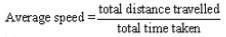(ii) Uniform speed refers to the constant speed of a moving body.  A body has a uniform speed if it travels equal distance in equal intervals of time, no matter how small these time interval may be.

Question 37:
(a) What is meant by the term ‘acceleration’ ? State the SI unit of acceleration.
(b) Define the term ‘uniform acceleration’. Give one example of a uniformly accelerated motion.
Solution :
(a) Velocity of a body is the distance travelled by it per unit time in a given direction. SI unit of velocity is m/s.
( b)(i)Speed is a scalar quantity whereas velocity is a vector quantity.
(ii) Speed of a body is distance travelled by it per unit time whereas velocity of a body is the distance travelled by it per unit time in a given direction.
(iii)Speed is always positive whereas velocity can be both positive as well as negative.
(c) Speed = 54km/h = 54 x (1000/3600) = 15m/s

Question 38:
The distance between Delhi and Agra is 200 km. A train travels the first 100 km at a speed of 50 km/h. How fast must the train travel the next 100 km, so as to average 70 km/h for the whole journey ?
Solution :
(a) Acceleration of a body is defined as the rate of change of its velocity with time. SI unit of acceleration is m/s2.
(b) A body has uniform acceleration if it travels in a straight line and its velocity increases by equal amounts in equal intervals of time. For example: Motion of a freely falling body.

Question 39:
A train travels the first 15 km at a uniform speed of 30 km/h; the next 75 km at a uniform speed of 50 km/h; and the last 10 km at a uniform speed of 20 km/h. Calculate the average speed for the entire train journey.

Solution :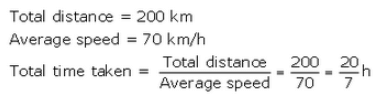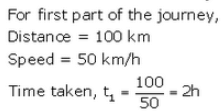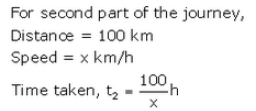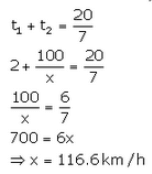Question 40:
A train travels the first 15 km at a uniform speed of 30 km/h; the next 75 km at a uniform speed of 50 km/h; and the last 10 km at a uniform speed of 20 km/h. Calculate the average speed for the entire train journey.
Solution :

(i) In the first part, train travels at a speed of 30 km/h for a distance of 15 km.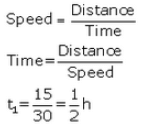(ii) In the second part, train travel at a speed of 50 km/h for a distance of 75 km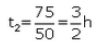(iii) in the third part, train travel at a speed of 20 km/h for a distance of 10 km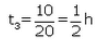Total distance covered  = 15+75+10 = 100km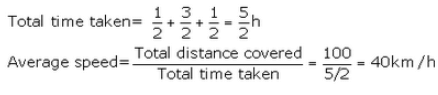Question 41:
A car is moving along a straight road at a steady speed. It travels 150 m in 5 seconds :
(a) What is its average speed ?
(b) How far does it travel in 1 second ?
(c) How far does it travel in 6 seconds ?
(d) How long does it take to travel 240 m ?
Solution :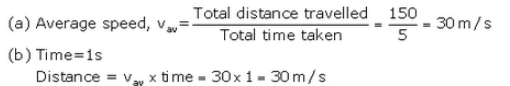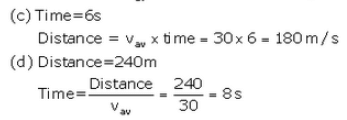Page No - 22

Question 55:
A body is moving along a circular path of radius R. What will be the distance travelled and displacement of the body when it completes half a revolution ?
Solution :
Distance travelled in half a rotation of a circular path is equal to the circumference of semi-circle, i.e.,

Distance travelled in half of a circular path is equal to the circumference of semi-circle, i.e = πR.

Displacement = diameter of circle = 2R

Question 56:
If on a round trip you travel 6 km and then arrive back home :
(a) What distance have you travelled ?
(b) What is your final displacement ?

Solution :
(i) Distance travelled = 6 km
(ii) Displacement = zero(since final position is same as initial position)

Question 57:
A body travels a distance of 3 km towards East, then 4 km towards North and finally 9 km towards East.
(i) What is the total distance travelled ?
(ii) What is the resultant displacement ?

Solution :
(i) Total distance travelled= 3 + 4 +9=16 km
(ii) The body travels a total distance of 12 km in east direction i.e. towards x-axis.
And it travels a distance of 4 km in North direction, i.e. towards y-axis. Hence, resultant displacement is

(i) Total distance travelled  = 3+4+9 = 16 km

(ii) The body travel a total distance of 12 km in east direction i.e toward  x-axis

And it travel a distance of 4 km in North direction, i.e toward  y- axis

Hence, resultant displacement is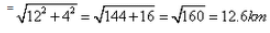Question 58:
A boy walks from his classroom to the bookshop along a straight corridor towards North. He covers a distance of 20 m in 25 seconds to reach the bookshop. After buying a book, he travels the same distance in the same time to reach back in the classroom. Find (a) average speed, and (b) average velocity, of the boy.
Solution :
(a) Total distance covered in going to the bookshop and coming back to the classroom = 20 + 20 = 40m
Total time taken= 25 + 25 = 50 sec

(a) total distance covered in going to the bookshop and coming back to the classrooom = 20+20 = 40 km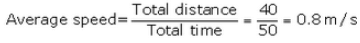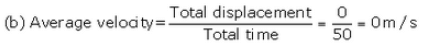Question 59:
A car travels 100 km at a speed of 60 km/h and returns with a speed of 40 km/h. Calculate the average speed for the whole journey.
Solution :
In the first case, car travels at a speed of 60 km/h for a distance of 100 km
In the second case, car travels at a speed of 40 km/h for a distance of 100 km
Total distance travelled = 200 km

In the first case , car travels at a speed of 60 km/h for a distance of 100 km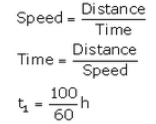In the second case, car travels at a speed of 40 km/h for a distance of 100km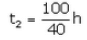Total distance travelled  = 200 km

Average speed=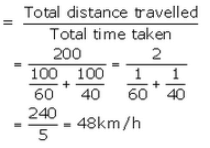Question 60:
A ball hits a wall horizontally at 6.0 m s-1. It rebounds horizontally at 4.4 m s-1. The ball is in contact with the wall for 0.040 s. What is the acceleration of the ball ?
Solution :
Initial velocity, u=6m/s
Final velocity ,v=-4.4m/s(the ball rebounds in opposite direction)
Time, t = 0.040 s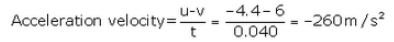The document Lakhmir Singh & Manjit Kaur: Motion, Solutions- 2 | Science Class 9 is a part of the Class 9 Course Science Class 9.
All you need of Class 9 at this link: Class 9

## Science Class 9

66 videos|355 docs|97 tests

## FAQs on Lakhmir Singh & Manjit Kaur: Motion, Solutions- 2 - Science Class 9

 1. What is motion?Ans. Motion is the change in position of an object with respect to its surroundings. It can be described in terms of distance traveled, speed, velocity, and acceleration.
 2. What are the different types of motion?Ans. There are different types of motion, including linear motion (motion in a straight line), circular motion (motion along a circular path), and rotational motion (motion around an axis).
 3. How is speed different from velocity?Ans. Speed is a scalar quantity that refers to the rate at which an object covers distance. Velocity, on the other hand, is a vector quantity that includes the speed of an object as well as its direction.
 4. What is meant by acceleration?Ans. Acceleration refers to the rate at which an object's velocity changes. It can be positive (increasing speed), negative (decreasing speed), or zero (constant speed).
 5. How are distance and displacement different?Ans. Distance is the total length of the path traveled by an object, while displacement is the shortest distance between the initial and final positions of the object. Displacement also takes into account the direction of motion.

## Science Class 9

66 videos|355 docs|97 testsExplore Courses for Class 9 examSignup to see your scores go up within 7 days! Learn & Practice with 1000+ FREE Notes, Videos & Tests.
10M+ students study on EduRev
Track your progress, build streaks, highlight & save important lessons and more!
Related Searches

,

,

,

,

,

,

,

,

,

,

,

,

,

,

,

,

,

,

,

,

,

,

,

,

;